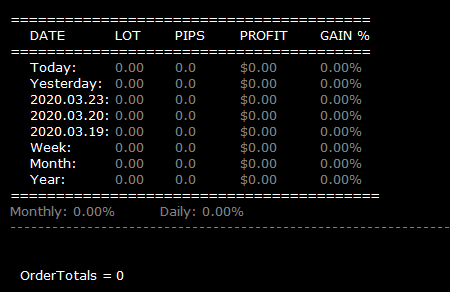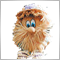# Converting Order totals from MT4 indicator to MT5 -- Need help356

Hi..

I am creating a dashboard for my totals.

I have 99% of it working.  Just having issues with the OrdersTotal for the daily and weekly figures.

```void DrawCurrentTrades() {
//+------------------------------------------------------------------+

double slPip, tpPip;
double allTPPips, allSLPips = 0;
double maxLoss, maxProfit = 0;
color c = White;
string text = "";
int colWidth1 = 200;

for (int i = OrdersTotal(); i >= 0; i--)

if ( IsValidOrder() )

// {

slPip = 0;
tpPip = 0;
buyProfit += OrderProfit() + OrderSwap() + OrderCommission();
buyPip += point2pip(MarketInfo(OrderSymbol(), MODE_BID) - OrderOpenPrice(), OrderSymbol());

if (OrderStopLoss() > 0.0) slPip = point2pip(OrderOpenPrice() - OrderStopLoss(), OrderSymbol());
if (OrderTakeProfit() > 0.0) tpPip = point2pip(OrderTakeProfit() - OrderOpenPrice(), OrderSymbol());

} else if (OrderType() == OP_SELL) {
sellCount++;
sellProfit += OrderProfit() + OrderSwap() + OrderCommission();
sellLot += OrderLots();
sellPip += point2pip(OrderOpenPrice() - MarketInfo(OrderSymbol(), MODE_BID), OrderSymbol());

if (OrderStopLoss() > 0.0) slPip = point2pip(OrderStopLoss() - OrderOpenPrice(), OrderSymbol());
if (OrderTakeProfit() > 0.0) tpPip = point2pip(OrderOpenPrice() - OrderTakeProfit(), OrderSymbol());
}
if (slPip != 0) {
maxLoss -= pip2money(slPip, OrderLots(), OrderSymbol());
allSLPips -= slPip;

}

if (tpPip != 0) {
maxProfit += pip2money(tpPip, OrderLots(), OrderSymbol()) + OrderSwap() + OrderCommission();
allTPPips += tpPip;
}
DrawText(0, 30, 20, "OrderTotals = "+ OrdersTotal(), White, FontSize);
//     }
```

this is what is should look likeBut should have numbers in the fields..

pls helpModerator
182451

Example: counting all POSITIONS (for all characters, for all Magic number)

```//+------------------------------------------------------------------+
//| Calculate all positions                                          |
//+------------------------------------------------------------------+
int CalculateAllPositions()
{
int total=0;

for(int i=PositionsTotal()-1; i>=0; i--)
if(m_position.SelectByIndex(i)) // selects the position by index for further access to its properties
total++;
//---
return(total);
}```356

Example: counting all POSITIONS (for all characters, for all Magic number)

Do I replace the whole section or just add  that to the top?Moderator
182451

Kristina Suh :

Do I replace the whole section or just add  that to the top?

The function itself (CalculateAllPositions ()) should be BELOW OnInit, OnTick, OnTradeTransaction.

A function call (CalculateAllPositions ()) is usually made from OnTick.Moderator
182451

### Example: Calculate Positions and Pending Orders356

### Example: Calculate Positions and Pending Orders

HI,

looks great.. I will try that.

Will the coding work on an Indicator?Moderator
182451

Kristina Suh :

HI,

looks great.. I will try that.

Will the coding work on an Indicator?

You know, sometimes I like to check - sometimes checking an idea is faster than waiting for an answer :)356

You know, sometimes I like to check - sometimes checking an idea is faster than waiting for an answer :)

HI.

It looks like some of the coding doesn't work on my Indicators.

What I am trying to do is display profits per day for the week and the month.

I'm still trying to work that out from your sent me.

I will try and bring some of the indicator into the EA.   and see how that goes.